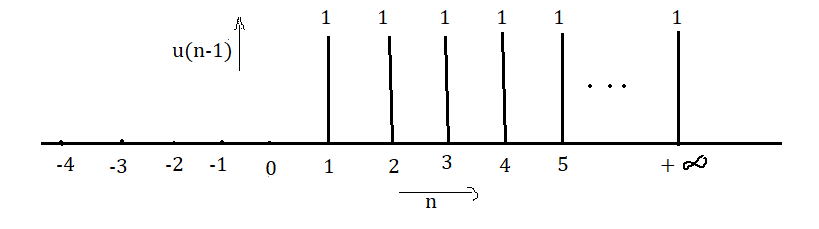### REPRESENTATION OF DISCRETE TIME SIGNALS - FUNCTIONAL & GRAPHICAL

Here you would see how to represent a discrete time DT signal in graphical as well as functional form by knowing the range of n - REPRESENTATION OF DISCRETE TIME SIGNALS - FUNCTIONAL & GRAPHICAL

REPRESENTATION OF DISCRETE TIME SIGNALS - FUNCTIONAL & GRAPHICAL

For this, you have to remember the functional representation of Unit-Step function u(n) which is

u(n) =  1,   for (n) ≥ 0

and                  u(n) =  0,   for (n) < 0

The above functional representation indicates that the value of u(n) is equal to 1 for n ≥ 0 and its value is equal to 0 for n < 0.

Its graphical representation is shown in Fig. 1.Fig. 1

Now, functional and graphical representation of any discrete time signal can be shown in the same way as above. The only thing you need to know is the range of ‘n’ for that particular signal. Let’s take some example:

## Example 1 : Functional and graphical representation of u(n-1)

For functional representation, write the function in the same way as written in the case of u(n). i.e.,

u(n-1) =  1,   for (n-1) 0   =>for n 1

and                  u(n-1) =  0,   for (n-1) < 0   => for n < 1

The functional representation of u(n-1) indicates that its value is equal to 1 for n ≥ 1 and its value is equal to 0 for n < 1.

Therefore, graphical representation of u(n-1) is shown in Fig. 2.Fig. 2

## Example 2: Functional and graphical representation of u(-n-2)

Functional representation of u(-n-2) would be

u(-n-2) =  1,      for (-n-2) 0   => -n 2     => n ≤ -2

and                  u(-n-2) =  0,      for (-n-2) < 0   => -n < 2     => n > -2

The functional representation of u(-n-2) indicates that its value is equal to 1 for all values of n less than or equal to -2  (i.e., n ≤ -2) and its value is equal to 0 for all values of n greater than -2 (i.e., n > -2).

Therefore, graphical representation of u(-n-2) would be

Hence Functional and graphical representation of any discrete time signal can be formed by finding the range of its value of n.

Hope this post on "REPRESENTATION OF DISCRETE TIME SIGNALS - FUNCTIONAL & GRAPHICAL" can help to clear doubts on how to represent any DT signal in functional and graphical form.

Click here to find VIDEO on Functional and Graphical representation of Discrete Time Signal

 You may also like: Circular Convolution with Zero Padding